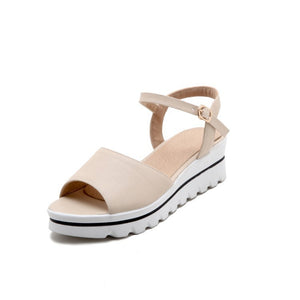# Open Toe Women Sandals Wedge Heels Shoes for Summer 8402

Regular price \$32.30 Sale price \$74.70

Heel Height: 4.5 cm
Platform Height: 2 cm

Size Note: We send CN size, if your foot is a little wide and fat, we suggest you choose 1 size larger.

Size Guide:
Euro/CN 34 = US 3 = 22cm (Foot width=8-8.5cm)
Euro/CN 35 = US 4 = 22.5cm (Foot width=8.5cm)
Euro/CN 36 = US 5 = 23cm (Foot width=8.5-9cm
Euro/CN 37 = US 6 = 23.5cm (Foot width=9cm)
Euro/CN 38 = US 7 = 24m (Foot width=9-9.5cm)
Euro/CN 39 = US 8 = 24.5cm (Foot width=9.5cm)
Euro/CN 40 = US 9 = 25cm (Foot width=9.5-10cm)
Euro/CN 41 = US 10 = 25.5cm (Foot width=10cm)
Euro/CN 42= US 11 = 26cm (Foot width=10-10.5cm)
Euro/CN 43 = US 12 = 26.5cm (Foot width=10.5cm)
Euro/CN 44= US 13 = 27cm (Foot width=10.5-11cm)
Euro/CN 45 = US 14 = 27.5cm (Foot width=11.5cm)
Euro/CN 46= US 15 = 28cm (Foot width=11.5-12cm)
Euro/CN 47 = US 16 = 28.5cm (Foot width=12.5cm)
Euro/CN 48= US 17 = 29cm (Foot width=12.5-13cm)